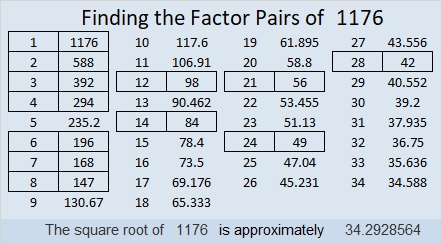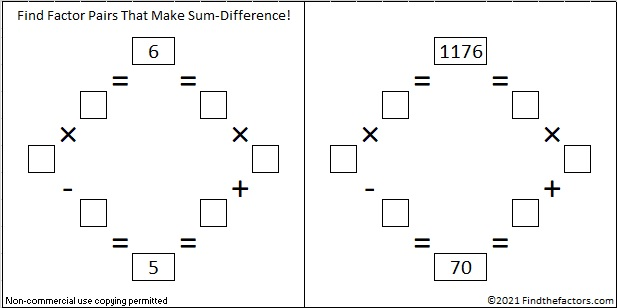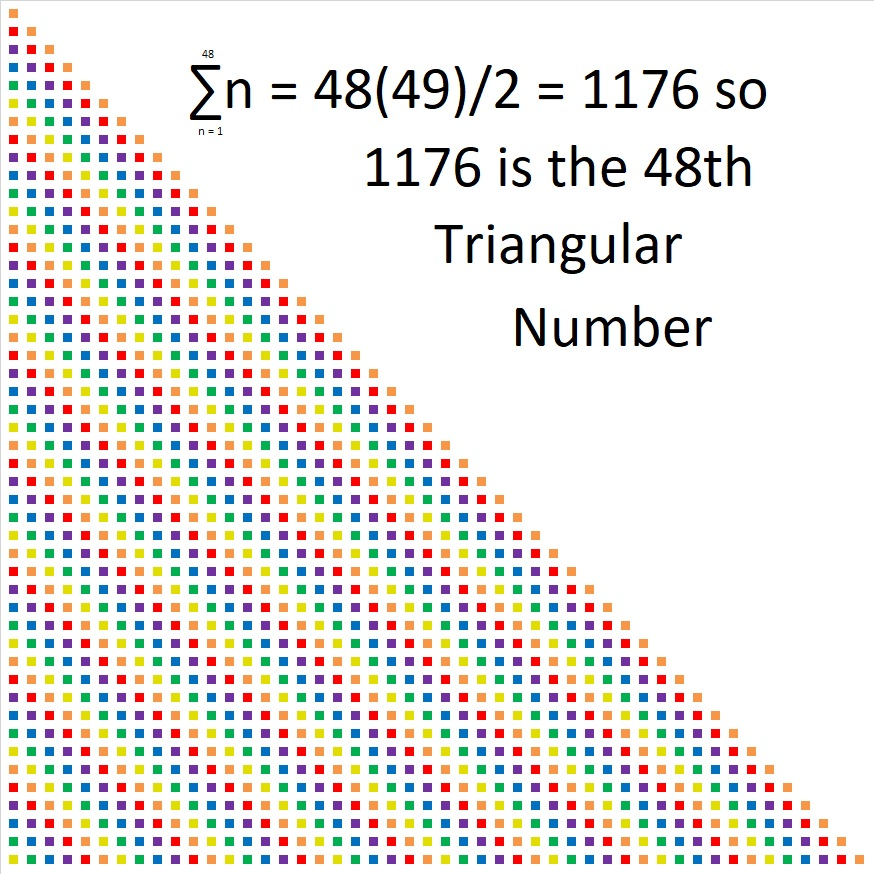# How to Simplify √1176

Contents

### Simplifying √1176:

About 83% of numbers whose square root can be simplified are divisible by 4 or by 9 or by both. It’s easy to see rather quickly if a number is divisible by either of them. I want to find square roots by doing as few divisions as possible. It is easier to divide a number by 4 than it is to divide it by 2 twice. Likewise, it is easier to divide a number by 9 than it is to divide it by 3 twice.

1176 is divisible by 4 because the number formed from its last two digits, 76, is divisible by 4. So let’s do that division:
1176÷4=294.

Can we divide 294 by 4? We can quickly see that 94 is not divisible by 4, so 294 is not divisible by 4. Let’s check to see if it is divisible by nine: 2 + 9 + 4 = 15, which is not divisible by 9 but is by 3. That means that 294 is not divisible by 9, but it is divisible by 3.

Since 294 is an even number that is divisible by 3, I will save time and make fewer mistakes if I just divide it by 6 instead of by 2 and then by 3.
294÷6 = 49

49 is a perfect square so I know I’ve done enough dividing for this particular number. I’ve made a gif to illustrate the steps I’ve just explained:make science GIFs like this at MakeaGif

### Factor Trees for 1176:

By doing just a little bit of arithmetic in my head, I can also tell by looking at that cake that the prime factorization of 1176 is 2³ × 3 × 7².

Some people still prefer to use factor trees to find square roots. Here are a few of its MANY possible trees.### Factors of 1176:

• 1176 is a composite number.
• Prime factorization: 1176 = 2 × 2 × 2 × 3 × 7 × 7, which can be written 1176 = 2³ × 3 × 7²
• The exponents in the prime factorization are 3, 1 and 2. Adding one to each and multiplying we get (3 + 1)(1 + 1)(2 + 1) = 4 × 2 × 3 = 24. Therefore 1176 has exactly 24 factors.
• Factors of 1176: 1, 2, 3, 4, 6, 7, 8, 12, 14, 21, 24, 28, 42, 49, 56, 84, 98, 147, 168, 196, 294, 392, 588, 1176
• Factor pairs: 1176 = 1 × 1176, 2 × 588, 3 × 392, 4 × 294, 6 × 196, 7 × 168, 8 × 147, 12 × 98, 14 × 84, 21 × 56, 24 × 49 or 28 × 42
• Taking the factor pair with the largest square number factor, we get √1176 = (√196)(√6) = 14√6 ≈ 34.29286### Sum-Difference Puzzles:

6 has two factor pairs. One of those pairs adds up to 5, and the other one subtracts to 5. Put the factors in the appropriate boxes in the first puzzle.

1176 has twelve factor pairs. One of the factor pairs adds up to ­70, and a different one subtracts to 70. If you can identify those factor pairs, then you can solve the second puzzle!The second puzzle is really just the first puzzle in disguise. Why would I say that?

### More about the Number 1176:

1176 is the sum of the first 48 numbers so we say it the 48th triangular number. We know it is the sum of the first 48 numbers because (48 × 49)/2 = 11761176 looks interesting in a few other bases:
It’s 3300 in BASE 7 because 3(7³ + 7²) = 3(392) = 1176,
6C6 in BASE 13 (C is 12 base 10) because 6(13²) + 12(13) + 6(1) = 1176,
600 in BASE 14 because 6(14²) = 1176,
1M1 in BASE 25 (M is 22 base 10) because 25² + 22(25) + 1 = 1176
and it looks like one of its factors, 196, in BASE 30
because 1(30²) + 9(30) + 6(1) = 1176

This site uses Akismet to reduce spam. Learn how your comment data is processed.